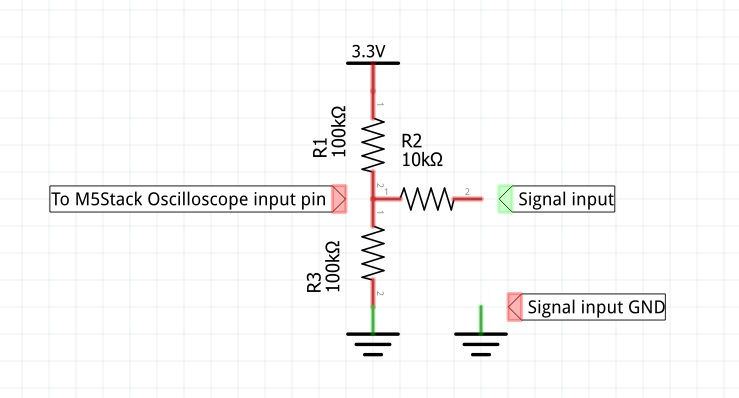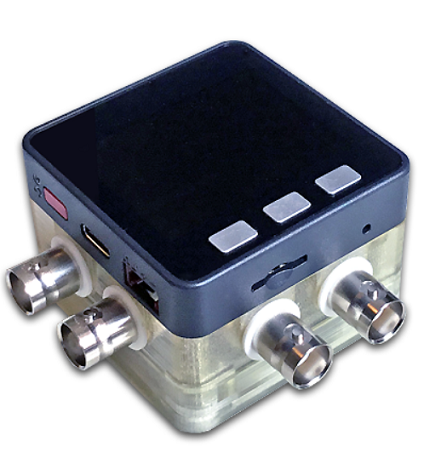# M5Stack 2 Channel Oscilloscope

• If the DAC input was damaged, it won't pick up any signal that you feed to it...

• Btw... check out the new development: http://forum.m5stack.com/topic/165/m5stack-multiapp-firmware

• If I understand this correctly, the ESP32 analog input captures only positive voltage.
How do I get the full -/+ range in there?

• Make a voltage divider with 2 resistors (100k or so...) with one end connected to GND and the other end to 3.3v.
The middle of the divider connected to oscilloscope input pin.
Apply input signal to input pin and you get +/- 1.65v input range.
I designed the code to be used with this divider, that's why, without the divider, the oscilloscope line is at the bottom of the screen.
Once you connect the divider, the signal line will be at the middle of the screen just like on a normal oscilloscope.

• Without the voltage divider, the amplitude is the same, just offset by 1.65v?

• The max amplitude is 3.3v. With the divider, the amplitude will be -1.65v to +1.65v (total 3.3v) The aplitude can be solved easy with a series of resistors at the imput. To change the input aplitude, replace the 10K resistor on the example below:• he amplitude will be -0.65v to +0.65v (total 3.3v)

@calin, I think you meant the input signal range will be -1.65v to +1.65v (total 3.3v). :)

• he amplitude will be -0.65v to +0.65v (total 3.3v)

@calin, I think you meant the input signal range will be -1.65v to +1.65v (total 3.3v). :)

yes. sorry for typo... edited now :)

• Awesome oscilloscope case - designed by Macsbug - infos here• This post is deleted!